Skip to main content

# Non-intrusive load monitoring and decomposition method based on decision tree

## Abstract

In order to realize the problems of non-intrusive load monitoring and decomposition (NILMD) from two aspects of load identification and load decomposition, based on the load characteristics of the database, this paper firstly analyzes and identifies the equipment composition of mixed electrical equipment group by using the load decision tree algorithm. Then, a 0–1 programming model for the equipment status identification is established, and the Particle Swarm Optimization (PSO) is used to solve the model for equipment state recognition, and the equipment operating state in the equipment group is identified. Finally, a simulation experiment is carried out for the partial data of Question A in the 6th “teddy cup” data mining challenge competition.

## Introduction

In recent years, non-intrusive power load monitoring and decomposition (NILMD) technology has attracted the attention of many scholars due to the high cost, low efficiency and limited application of traditional power load monitoring methods . Har  initially put forward the idea and theory of non-invasive load decomposition, mainly through load decomposition at the entrance of residential electricity load. Roos et al.  proposed multi-level neural network algorithm to analyze power load characteristics. Drenker et al.  developed a database system which can extract the steady-state load characteristics of electrical equipment. The system determines the energy consumption of individual appliances being turned on and off within a whole building’s electric load. By using changes in active power and reactive power, they used clustering analysis algorithm to identify electrical equipment. To improve the recognition effect of equipment Laughmam et al.  used the FFT algorithm to analyze the characteristics of harmonic load on this basis. Suzuki et al.  used integer programming to decompose and identify electrical equipment. Choksi et al.  proposed to identify electrical equipment based on power load characteristics and decision tree algorithm. Hassan et al.  expands and evaluates appliance load signatures based on V-I trajectory—the mutual locus of instantaneous voltage and current waveforms, and they also demonstrate the use of variants of differential evolution as a novel strategy for selection of optimal load models. Lu et al.  proposed a classification method based on extreme learning machine (ELM) algorithm for electricity consumption behavior analysis, and the feature preference strategy is adopted to extract the best feature sets of the load curve, which were used as the input of ELM network. However, the above non-invasive equipment identification algorithms only consider the load data at the load entrance, so it cannot achieve high-precision identification through a single identification algorithm. This load decomposition technique is expected to be a better technique for dynamic load separation because it includes transient and steady-state characteristics to achieve better energy saving and emission reduction effects in the future. Including multiple features in the feature matrix helps to increase computational time and complexity. We can potentially reduce computing time and complexity by using specific features of specific categories of devices. Avoiding unnecessary extraction is helpful to the training of database and the optimization of decomposition and recognition technology. In this paper, event detection algorithm, load decision tree algorithm and 0–1 quadratic programming model are combined to improve the accuracy of power load identification.

## Related work

The following methods are used to realize our research in NILMD system.

### Event detection algorithm

Event detection  and load characteristics are mutually complementary. This paper takes the change value Δp of the characteristic value p of active power as the criterion for event detection, and sets a reasonable power change threshold according to the electrical equipment and operating parameters. However, some electrical equipment will have a large peak of power at the moment of starting (the motor starting current is higher than the rated current). Although this does not affect the accuracy of determining the time of occurrence of the event, it may cause inaccurate change of the steady-state power of the electrical equipment. The transient process of different equipment is long to short, so it is necessary to combine the data within a certain time range to determine whether an event has occurred. Due to the power quality (such as voltage drop), the active power will change suddenly and it is easy to make wrong judgment. In the case that the equipment group contains both the low power and the high power equipment, if the threshold setting is too large, the high power equipment will cover the low power equipment, and if the threshold setting is too small, the number of detected events will be multiplied. Therefore, the threshold setting must consider both the power level of the equipment contained in the equipment group and the change value of the steady state power. In this paper, using time as horizontal axis and power value as vertical axis, the time-power diagram is drawn, and the power threshold value is determined by observing the graph and calculating the percentage of the power value of electrical equipment. Take equipment group 4, 5 and 6 in Annex 3 of question A as examples to determine the power threshold as in Table 1.

The steps of the event detection algorithm are as follows.

Step 1. Calculate the difference $$\Delta p_{t}$$ between the current time of active power and the previous time. If $$\Delta p _{t}\ge p_{1}$$, go to Step 3, otherwise enter Step 2.

Step 2. Read the next time data and return to Step 1.

Step 3. The event duration D increases by 1 second on its original basis and go to Step 4.

Step 4. Read the next time data and calculate to get $$\Delta p_{t+D}=p_{t+D}-p_{t}$$. If $$\Delta p_{t+D}\ge p_{1}$$, go to Step 5, otherwise, go to Step 6.

Step 5. Read the next time data and return to Step 3.

Step 6. According to the event duration D, we can get the end time of the event is $$t+D$$. Calculate the change value of active power before and after the event is calculated. If $$\Delta p_{t+D} \ge p_{2}$$, go to Step 7, otherwise, it will be judged that no events have occurred, return to Step 2.

Step 7. Output results. According to the positive and negative conditions of $$\Delta p_{t+D}$$, we can judge whether this event is a power increase event or a power decrease event. If the result is positive, the active power of the system increases. We judge that it is an ascending event, which is generally caused by the start of operation or the change of state of the electrical equipment. If the result is negative, it indicates that the active power of the system decreases. It is judged as a falling event, which is generally caused by the change of the running state of the electrical equipment when it is cut off. We think of time $$t+D$$ as the end of the event, and the time as the beginning of the next event. In order to reflect the change of power more objectively, we took the active power data within five seconds before time t. The arithmetic mean value is taken as the active power of the system before the event occurs. Similarly, the active power data of five seconds after $$t+D$$ time are taken, and the arithmetic average value represents the active power of the system after the event. Therefore, we get the difference between the two, which is the required active power variation $$\Delta p_{t+D}$$.

Taking equipment group 4 as an example, we use the event detection algorithm to find the moment when the running state of the equipment changes, as shown in Fig. 1.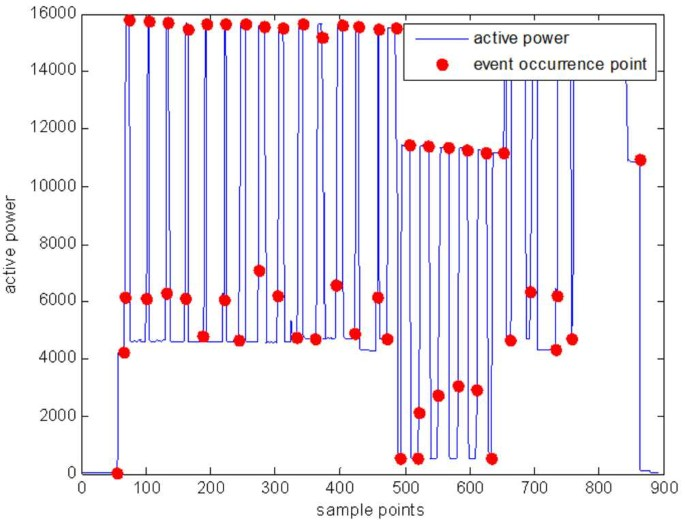Figure 1

By setting a reasonable power change threshold, the event detection algorithm can identify load events with large active power variation value and determine the occurrence point of the event. Thus, the event detection algorithm is of great help to analyze the running state of each electrical equipment. In this paper, the event detection algorithm is used to segment the running state of the equipment group, and then the decision tree algorithm is used to identify the electrical equipment.

### Load decision tree algorithm for equipment composition identification

The load decision tree algorithm is similar to the load decomposition algorithm. The load identification algorithm also compares the extracted unknown load characteristic parameters with the known load characteristic parameters in the database, and then finds the known load closest to the extracted load characteristic parameters as the identification result. Therefore, we need to make decision tree load identification on the basis of load database. In this paper, the load decision tree algorithm  is based on three load databases (active power and reactive power in different states of the equipment, the harmonic content amplitude database, and the V-I trajectory of the load). The recognition algorithm based on decision tree requires relatively little computation, so it can avoid using low-power load characteristics for identification to some extent. This division of data leads to reduced computational complexity and time, and it is considered a better algorithm when it comes to multi-label classification problems. Now we introduce the decision tree algorithm into the load identification of our electrical equipment. The flow chart of the decision tree load identification algorithm is shown in Fig. 2.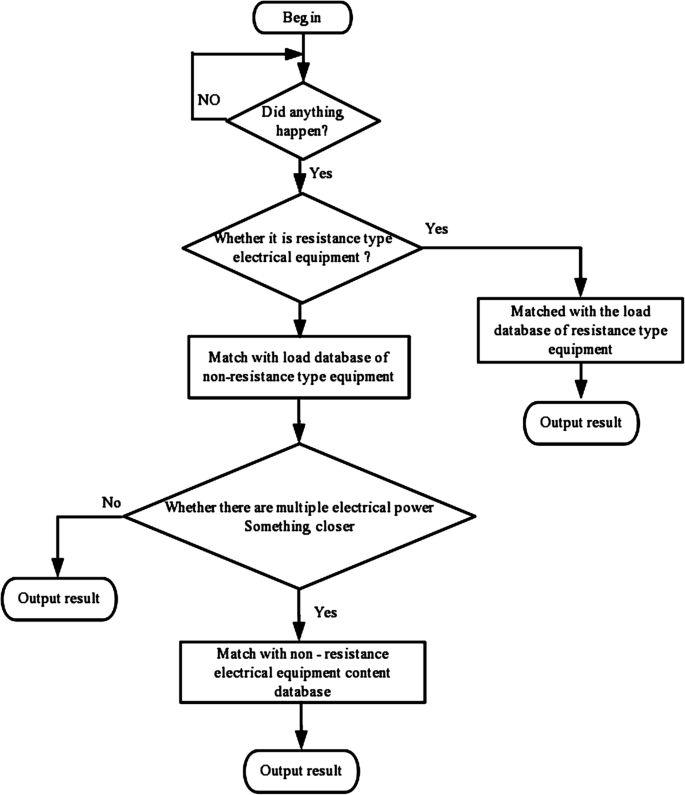Figure 2

The steps of decision tree algorithm for load identification are as follows.

Step 1. The event detection algorithm determines whether the load change event occurs, if not, enter Step 2, otherwise, enter Step 3.

Step 2. Read the next time data and return to Step 1.

Step 3. Determine whether the equipment in which the event occurred is pure resistive. If it is pure resistive electrical equipment, Step 4 should be followed, otherwise, go to Step 5.

Step 4. Compare with the pure resistive electrical equipment power database. Since the event equipment is pure resistive, only the active power needs to be compared.

Step 5. Output the equipment with the most similar active power as the identification result.

Step 6. Compared with non-pure resistance equipment power database.

Step 7. Determine if there are many similar equipment in the Step 6. If not, go to Step 8; otherwise, enter Step 9.

Step 8. Output the equipment with the most similar active power in Step 6 as the identification result.

Step 9. The V-I trajectories of event loads are extracted, and compared it with the harmonic content database.

Step 10. Output the equipment with the most similar harmonic content in Step 9 as the identification result.

The matching in Step 4, Step 6 and Step 9 is based on the Euclidean distance. The eigenvalue of the event load is regarded as a point in the Euclidean space, and the eigenvalue in the database is regarded as a point in the space. Point $$x=(x_{1},x_{2}, \dots ,x_{n})$$ and $$y=(y_{1},y_{2},\dots ,y_{n})$$ respectively represent the extracted eigenvalues and the eigenvalues in the database, we use (1) to represent the approximate degree between the two points. The smaller the value is, the higher the approximate degree is

$$d(x,y)=\frac{\sqrt{\sum_{i=1}^{n}{(x_{i}-y_{i})}^{2}}}{\sqrt{x _{1}^{2}+\cdots +x_{p}n^{2}}}.$$
(1)

To judge whether the matching results are close in Step 7 means to compare them by using (1) in Step 6. If the minimum results are less than δ (δ small enough), it is considered to be close. Then, Step 9 uses harmonic content amplitude to identify.

As can be seen, if the equipment is an approximate pure resistance, the most effective load feature for its identification is the V-I trajectory. The load decision tree algorithm can first determine whether the load is pure resistance, which only needs to be compared with the load of resistance in the database, thus eliminating unnecessary comparison. In the process of comparing the feature parameters extracted by the identification algorithm with the database, it is impossible to accurately identify the unknown load if the situation is similar to many known loads. At this time, the unknown load can be further identified through other load characteristics. Although the previous load feature is not enough to get the final correct identification result, it can reduce the range of similarity comparison of feature parameters in the future.

### Establishment of 0–1 optimization model for equipment state identification

The load characteristic matrix of all equipment is calculated by the load characteristic of the database, and its load characteristic matrix is shown as

$$\varPsi = \begin{bmatrix} \varPsi _{11} & \ldots & \varPsi _{1N} \\ \vdots & \ldots & \vdots \\ \varPsi _{M1} & \ldots & \varPsi _{MN} \end{bmatrix}.$$
(2)

Where, $$N=\sum_{k=1}^{l}{N_{k}}$$, $$N_{k}$$ is the number of the state of equipment k, and l is the numbel of equipment. For electrical equipment with multiple working states, each working state is treated as an electrical equipment, that is, N will be greater than the actual number of electrical equipment. M is the number of load characteristics used in the identification algorithm

$$\varPsi _{ji}= [f_{1},f_{2},\dots ,f_{n} ]^{\mathrm{ T }}.$$
(3)

Where, $$\varPsi _{ji}$$ is the load characteristic vector of load characteristic j of equipment i, $$f_{i}$$ is the load characteristic data in the database, n is the number of the load characteristic j.

Extract characteristic vector $$Y'$$ from the measured data to be identified

\begin{aligned}& Y'= \bigl[y'_{1},y'_{2}, \dots ,y'_{M} \bigr]^{\mathrm{ T }}, \end{aligned}
(4)
\begin{aligned}& y'_{j}= [f_{1},f_{2},\dots ,f_{n} ]^{\mathrm{ T }}. \end{aligned}
(5)

Where, $$y'_{j}$$ is the load characteristic vector of load characteristic j extracted from the measured data.

The state vector

$$X= [x_{1},x_{2},\dots ,x_{N} ]^{\mathrm{ T }}.$$
(6)

Where, X is the state vector of load (0 means not in this state, 1 means in this state).

Through the above non-invasive load identification based on decision tree, we can know the state vector X when the equipment state changes. Then the load characteristic vector Y of this equipment can be known from the load characteristic database

$$Y= [y_{1},y_{2},\dots ,y_{M} ]^{\mathrm{ T }}= \varPsi X.$$
(7)

Where $$y_{j}$$ is the load characteristic vector of load characteristic j extracted from the load characteristic database.

We can get the relationship between $$Y'$$ and Y is as follows

$$Y'=Y+\varepsilon =\varPsi X+\varepsilon .$$
(8)

After the event detection algorithm detects the occurrence of an event, we extract the characteristic vector Y to be recognized. According to the established load characteristic database, the state vector X is solved to minimize the error ε. Where, $$Y'$$ is a redundant measurement. Thus, it is impossible to solve the problem directly based on (8) (If the error is not considered, there is no solution to (8) because the number of equations exceeds the number of unknowns), but an approximate solution of (8) can be found. The least squares method is used to transform the redundant equation into a minimum problem.

$$\min J={\varepsilon }^{\mathrm{ T }}\varepsilon .$$
(9)

Thus, problem (9) is transformed into a 0–1 quadratic programming problem, and its mathematical model is shown in (10).

\begin{aligned}& \min J={Y'}^{\mathrm{ T }}{Y'}-2{Y'}^{\mathrm{ T }} \varPsi X+ \frac{1}{2}\bigl(X\varPsi ^{\mathrm{ T }}2\varPsi X\bigr) \\& \quad \textstyle\begin{cases} \mbox{s.t. } \sum_{i=1}^{N_{k}}x_{i}=1, \\ \quad x_{i}=\{0,1\}. \end{cases}\displaystyle \end{aligned}
(10)

According to the relevant knowledge of linear algebra, it can be proved that $${\varPsi }^{\mathrm{ T }}2\varPsi$$ is a positive definite (or semi-positive definite) matrix. It can be seen that the objective function is strictly convex function (or convex function) and the feasible region is also a convex set. So we can get that the programming problem (10) is a convex programming problem. According to the theory of convex programming in nonlinear programming problem, problem (10) has the global optimal solution.

Problem (10) is a discrete problem. Most of the traditional methods for solving the discrete problems are combined algorithms, such as the Implicit Enumeration and the Exhaustive method. Although this kind of algorithm can accurately find the global optimal solution of the problem, its computational cost increases with the increase of the problem size. The other is discrete Heuristic Algorithm, such as Genetic Algorithm. The biggest disadvantage of this kind of algorithm is that it can not deal with constraints well and it is easy to premature convergence. However, there is no such problem in the continuous method, so the above problems are transformed into the continuous method to solve them. The equivalent model of its continuity constraint is shown in (11).

\begin{aligned}& \min J={Y'}^{\mathrm{ T }}{Y'}-2{Y'}^{\mathrm{ T }} \varPsi X+\frac{1}{2}\bigl(X \varPsi ^{\mathrm{ T }}2\varPsi X\bigr). \\& \quad \textstyle\begin{cases} \mbox{s.t. } \sum_{i=1}^{N_{k}}x_{i}=1,k=1,2,\dots ,l, \\ \quad \sum_{i=1}^{N}(x_{i}-x_{i}^{2})=0. \end{cases}\displaystyle \end{aligned}
(11)

### Particle swarm optimization algorithm of 0–1 programming model for equipment state recognition

Particle Swarm Optimization (PSO) is an evolutionary computing technique proposed by Eberhart and Kennedy . It originates from the study of predation behavior of birds. Similar to genetic algorithms, PSO is an iterative optimization tool [16, 17].

Let’s say I have L particles in a population, and each particle is an individual in l dimensional $$R_{l}$$. Different individuals have different position $$x=(x_{1},x_{2},\dots ,x_{l})$$ and corresponding to different individual fitness function value $$F_{k}$$ are related to the objective function values. The specific steps are as follows.

Step 1. (Initialization) The state vector of each load is considered as a population. The population size Ñ, learning coefficient $$c_{1}$$ and cognitive coefficient $$c_{2}$$ is determined. We regard each load as a particle, and the position vector of the i load is $$x_{i}$$ and the velocity vector is $$v_{i}$$, $$i=1,2,\dots ,N$$. State vectors of N loads are randomly generated as initial population $$X(0)$$. Set the termination criteria. Let $$t=0$$.

Step 2. (Individual evaluation) Calculate the optimal fitness $$x_{pj}(t)$$ and global optimal fitness $$x_{gj}(t)$$ of each individual in the state vector $$X(t)$$. If the termination criteria is satisfied, output the current optimal, otherwise return to Step 3.

Step 3. (Update speed and position) Use (12) and (13) to update the speed and position of each load.

\begin{aligned}& v_{ij}(t+1)=v_{ij}(t)+c_{1}r_{1}(t) \bigl(x_{pj}(t)-x_{ij}(t)\bigr)+c_{2}r_{2}(t) \bigl(x _{gj}(t)-x_{ij}(t)\bigr), \end{aligned}
(12)
\begin{aligned}& x_{ij}(t+1)=x_{ij}(t)+v_{ij}(t+1). \end{aligned}
(13)

Where, $$v_{ij}(t)$$ is the speed vector of the i load before the update, $$v_{ij}(t+1)$$ is the speed vector of the i load after the update, $$x_{pj}(t)$$ is the individual optimal, $$x_{gj}(t)$$ is the global optimal, and $$x_{ij}(t)$$ is the position vector of the i load before the update.

Step 4. (Update state vector) Update the best position and the global optimal position of each load, and update the population.

Step 5. (Termination verification) If the termination criteria are met, the individual with the maximum fitness in output $$X(t+1)$$ is taken as the optimal solution and the calculation is terminated, otherwise, let $$t=t+1$$ and return to Step 2.

## Numerical experiment

Take the measurement data of equipment group 5 in Annex 3 of Question A as an example. We analyzed the voltage, current and other data of the entire line collected in equipment group 5. We identifies the electrical equipment composition of the equipment group, decomposes the running state of each equipment, and estimates the real-time power consumption.

The data used to support the findings of this study are available at the question A of the 6th “teddy cup” data mining challenge competition (http://www.tipdm.org/bdrace/tzjingsai/20170921/1253.html).

### Data description and preparation

NILMD device measured the voltage and current data on the entire line. They can be regarded as the superposition of voltage and current data of each electrical equipment. The measured data provided in the Annex of Question A has single state data and superposed state data. Based on the database of steady-state characteristic parameters (active power, reactive power, current harmonics, power factor, V-I trajectory) extracted from questions A(1) and A(2), this paper conducts power load identification and decomposition for multi-equipment questions A(3) and A(4).

According to the current, active power, reactive power and other data of the electrical equipment, the order is sorted from first to last, from small to large. We select the three ON/OFF state equipment of the Question A equipment YD3, YD5, and YD11, and divide and label each state of the equipment, as shown in Table 2.

### Numerical experiment process and results

Based on the database and the power threshold table, we will carry out event detection on the power data to be tested, which is shown in Fig. 3.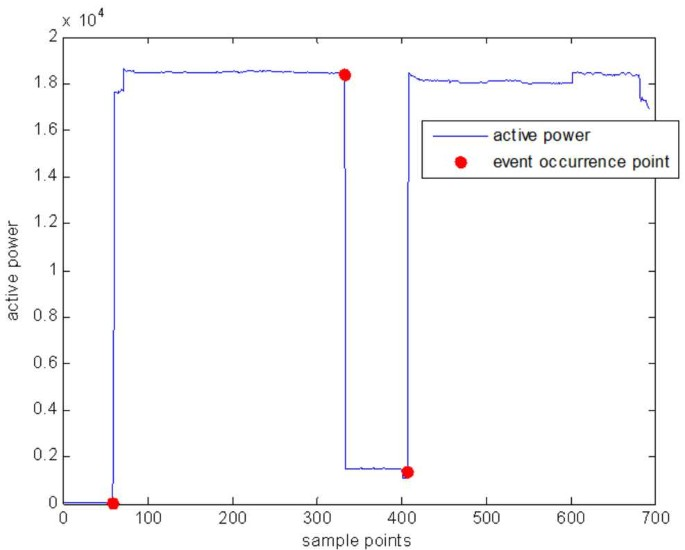Figure 3

We use the event detection algorithm to find out when the running state of the equipment changes. From Fig. 3, we can see the point in time when the event occurred. In this paper, after the running state of the equipment group is segmented, the load decision tree identification algorithm is used to identify the equipment composition of the equipment group.

The following is an explanation of the three load identification processes with representative significance in event detection.

Load opening event occurred in the 60th second, and the V-I trajectory is shown in Fig. 4. The result identified by the Step 3 of the load decision tree algorithm is the non-pure resistance class load, then the power data is compared with the non-pure resistance devices of YD1–YD11 devices in the database. We found the YD11 closest to the detected power variation characteristics. The equipment YD11 (Skyworth TV) was identified from the equipment group 5 to be tested.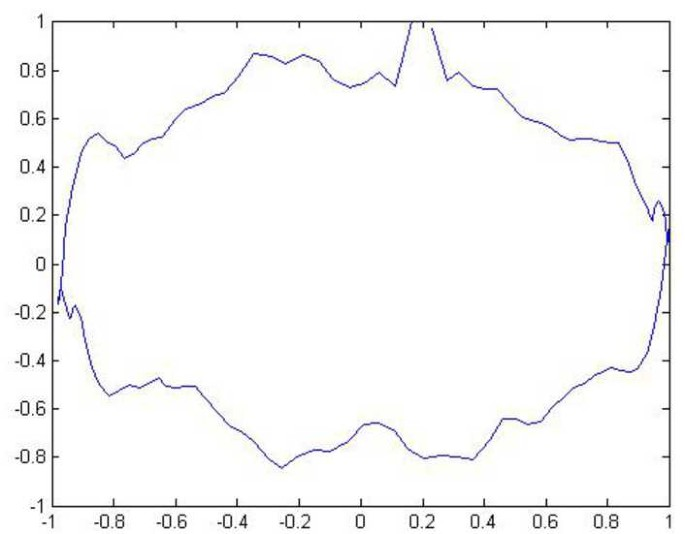Figure 4

At the second event point is at 339 seconds, we analyze the load event identification at the point. The V-I trajectory is shown in Fig. 5. The result we identified is the pure resistive load. Then it compares the power with the pure resistance equipment in the database, and we found that the equipment YD5 was the closest to the detected power change. Thus, the 339-second load event is identified as the YD5, that is, the equipment YD5 (incandescent lamp) is identified from the equipment group 5.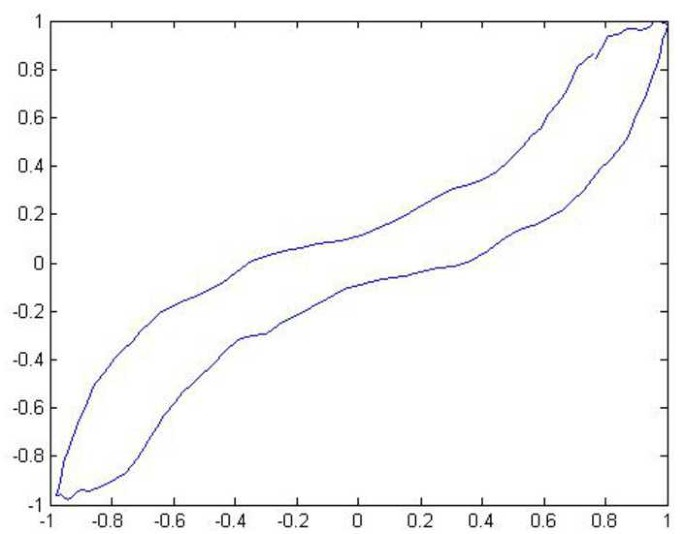Figure 5

At the third event point is at 405 second, the extracted V-I trajectory is shown in Fig. 6. The result of our identification is a pure resistance load, and then it compares the power with the pure resistance equipment in the database. The closest power change we can detect is the equipment YD3. Thus, the 405-second load event is identified as the YD3, that is, the equipment YD3 (Jiuyang hot kettle) is identified in the equipment group 5.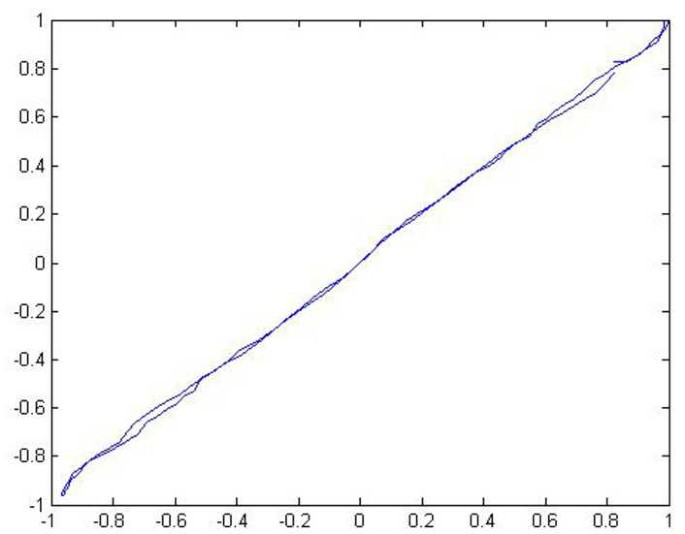Figure 6

We used the load decision tree algorithm to identify three equipment in the equipment group 5. the YD3 (Jiuyang hot kettle), the YD5 (incandescent lamp), and the YD11 (Skyworth TV). This exactly matches the actual results of the equipment composition given in Annex 3. Based on the known equipment composition of the equipment group 5, the 0–1 continuity quadratic programming model (see (11)) is used to identify the state of the YD3, YD5 and YD11.

In this paper, three kinds of load characteristics are extracted. active power characteristics (mean and variance) and reactive power characteristics (mean and variance), as shown in Table 3.

The equipment YD3, YD5 and YD11 are all ON/OFF equipment. Let $$N_{1}=N_{2}=N_{3}=2$$, $$N=6$$, and $$M=2$$ refers to the load characteristics of active and reactive power used in the identification algorithm. The state vector $$X=(x_{1},x_{2},x_{3},x_{4},x_{5},x_{6})^{\mathrm{ T }}$$, $$x_{i}=\{0,1\}$$, $$i=1,2,\dots ,6$$.

The power characteristic data of the equipment to be tested is shown in Table 4. Let’s take the first event as an example, $$Y'=(18\mbox{,}482.06,1545.28,375.81,10.77)^{\mathrm{ T }}$$.

The continuity method solves the equivalent model as shown in equation (14).

\begin{aligned}& \min J={Y'}^{\mathrm{ T }}{Y'}-2{Y'}^{\mathrm{ T }} \varPsi X+\frac{1}{2}\bigl(X \varPsi ^{\mathrm{ T }}2\varPsi X\bigr). \\& \quad \textstyle\begin{cases} \mbox{s.t. } x_{1}+x_{2}=1, \\ x_{3}+x_{4}=1, \\ x_{5}+x_{6}=1, \\ \sum_{i=1}^{6}(x_{i}-x_{i}^{2})=0. \end{cases}\displaystyle \end{aligned}
(14)

The results of the PSO for 0–1 programming are shown in Table 5.

We have completed data mining of non-invasive load decomposition. Due to the large data, some of the operation records and real-time power consumption of the equipment group 5 are shown in Table 6 and Table 7 respectively.

In this paper, a non-invasive power load decomposition and identification method is proposed, which integrates event detection algorithm, load decision tree algorithm and 0–1 quadratic programming model. Through numerical experiments, the algorithm in this paper is compared with the algorithms in other references, as shown in Table 8. We did the same experiment with other equipment groups data in Question A. The experimental results show that this method can effectively improve the accuracy of power load identification.

## Conclusion

The decision tree analysis method and 0–1 programming model are established in this paper. The algorithm can determine the state, operation and operation time of each electrical equipment. It can be seen from the analysis results that the algorithm in this paper has higher accuracy, higher anti-interference and stronger identification ability. NILMD technology based on decision tree has the advantages of easy operation, low cost (short payback period), high reliability, good data integrity and broad development prospects, which is of irreplaceable engineering significance. It is convenient for the residents to monitor the running state and the situation of electricity consumption. In addition, it can remind users to arrange electricity reasonably, adjust the difference between valley and peak electricity consumption, and reduce the damage of network line, so as to achieve the purpose of energy saving and consumption reduction.

## Abbreviations

PSO:

Particle Swarm Optimization

NILMD:

Non-intrusive Load Monitoring and Decomposition

$$N_{k}$$ :

the number of the state of equipment k

N :

the number of equipment

M :

the number of load characteristics used in the identification algorithm

$$\varPsi _{ji}$$ :

the load characteristic vector of load characteristic j of equipment i

$$f_{i}$$ :

the load characteristic data in the database; Extract characteristic vector $$Y'$$ from the measured data to be identified

X :

the state vector of load

Y :

this equipment can be known from the load characteristic database

## References

1. 1.

Hart GW. Noninstrusive appliance load monitoring. In: Proceedings of the IEEE. vol. 80. 1992. p. 1870–91.

2. 2.

Roos JG, Lan IE, Botha EC et al.. Using neural networks for non-intrusive monitoring of industrial electrical loads. In: Proceedings on instrumentation and measurement technology conference in Jpn, Hamamatsu. 1994. p. 1115–8.

3. 3.

Drenker S, Kader A. Nonintrusive monitoring of electric loads. IEEE Comput Applic Power. 1999;12(4):47–51.

4. 4.

Laughman C, Lee K, Cox R et al.. Power signature analysis. IEEE Power Energy Mag. 2003;1(2):56–63.

5. 5.

Suzuki K, Inagaki S, Suzuki T et al.. Non-intrusive appliance load monitoring based on integer programming. IEEJ Transactions on Power and Energy. 2008;128(11):1386–92.

6. 6.

Choksi KA, Jain SK. Pattern matrix and decision tree based technique for non-intrusive monitoring of home appliances. In: 2017 7th international conference on power systems (ICPS). Pune, India. New York: IEEE; 2017. p. 824–9.

7. 7.

Kim J, Le TT, Kim H. Non-intrusive load monitoring based on advanced deep learning and novel signature. Comput Intell Neurosci. 2017.

8. 8.

Hassan T, Javed F, Arshad N. An empirical investigation of V-I trajectory based load signatures for non-intrusive load monitoring. IEEE Trans Smart Grid. 2013;5(2):870–8.

9. 9.

Lu J, Chen ZM, Gong GJ, Xu ZQ, Qi B. Classification analysis method for electricity consumption behavior based on extreme learning machine algorithm. Autom Electr Power Syst. 2019;43:97–104.

10. 10.

Jiang B. A non-invasive residential load decomposition method based on deep learning. 2017.

11. 11.

Wang ZC. Research on non-invasive monitoring method of residential electricity load. 2015.

12. 12.

Yi J, Li ZD, Li H. Decision tree algorithm in non-invasive monitoring cell phone traffic. Comput Sci. 2016;A(1):361–4.

13. 13.

Yang Y. Non-invasive load identification based on decision tree. Sci Technol Innov. 2018;13:54–5.

14. 14.

Ruan L, Zheng X. Research and application of residential load characteristics. Shanghai University Of Electric Power; 2014.

15. 15.

Kennedy J, Eberhart R. Particle swarm optimization. In: Proc IEEE int conf on neural networks. Australia, Perth. New York: IEEE; 1995. p. 1942–8.

16. 16.

Xue F, Chen G, Gao S. Solving 0–1 integer programming problem by hybrid particle swarm optimization algorithm. Comput Technol Autom. 2011;1:86–9.

17. 17.

Sun Y, Gao YL. An adaptive particle swarm optimization algorithm for soling multi-objective 0–1 programming problem. Comput Appl Softw. 2009;2:71–2.

Download references

### Acknowledgements

At the point of finishing this paper, I’d like to express my sincere thanks to all those who have lent me hands in the course of my writing this paper. First of all, I’d like to take this opportunity to show my sincere gratitude to my supervisor, Mr Xianfeng Ding, who has given me so much useful advices on my writing, and has tried his best to improve my paper. Secondly, I’d like to express my gratitude to my classmates who offered me references and information on time. Without their help, it would be much harder for me to finish this paper.

### Availability of data and materials

The data used to support the findings of this study are available at the question A of the 6th “teddy cup” data mining challenge competition. (http://www.tipdm.org/bdrace/tzjingsai/20170921/1253.html). Please contact author for data requests.

## Funding

There is no funding for this research.

## Author information

Authors

### Contributions

JL mainly carried out algorithm research and writing manuscripts. XFD is mainly responsible for algorithm research and revising papers. After we received the referee’s comments, he did a good job of expanding the literature review and modifying the contents of Sect. 2.1 to provide a deeper understanding of the relevant publishing work. DQ is mainly responsible for supervising and software programming. HYL is a new member of my group and contributed much in the revised version of our manuscript. She revised the manuscript’s English language, formula and abbreviation. All authors read and approved the final manuscript.

### Corresponding author

Correspondence to Xianfeng Ding.

## Ethics declarations

### Competing interests

The authors declare that they have no competing interests.

## Additional information

### Publisher’s Note

Springer Nature remains neutral with regard to jurisdictional claims in published maps and institutional affiliations.

## Rights and permissions

Open Access This article is licensed under a Creative Commons Attribution 4.0 International License, which permits use, sharing, adaptation, distribution and reproduction in any medium or format, as long as you give appropriate credit to the original author(s) and the source, provide a link to the Creative Commons licence, and indicate if changes were made. The images or other third party material in this article are included in the article’s Creative Commons licence, unless indicated otherwise in a credit line to the material. If material is not included in the article’s Creative Commons licence and your intended use is not permitted by statutory regulation or exceeds the permitted use, you will need to obtain permission directly from the copyright holder. To view a copy of this licence, visit http://creativecommons.org/licenses/by/4.0/.

Reprints and Permissions